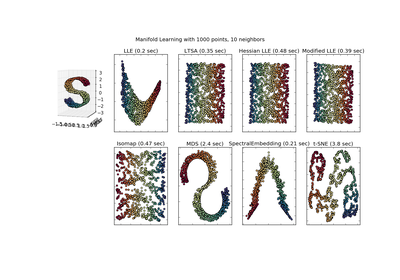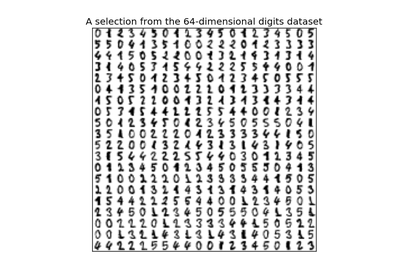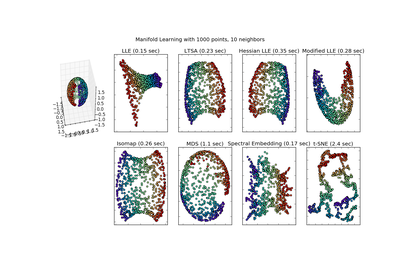# `sklearn.manifold`.Isomap¶

class `sklearn.manifold.``Isomap`(n_neighbors=5, n_components=2, eigen_solver='auto', tol=0, max_iter=None, path_method='auto', neighbors_algorithm='auto', n_jobs=1)[source]

Isomap Embedding

Non-linear dimensionality reduction through Isometric Mapping

Read more in the User Guide.

Parameters: n_neighbors : integer number of neighbors to consider for each point. n_components : integer number of coordinates for the manifold eigen_solver : [‘auto’|’arpack’|’dense’] ‘auto’ : Attempt to choose the most efficient solver for the given problem. ‘arpack’ : Use Arnoldi decomposition to find the eigenvalues and eigenvectors. ‘dense’ : Use a direct solver (i.e. LAPACK) for the eigenvalue decomposition. tol : float Convergence tolerance passed to arpack or lobpcg. not used if eigen_solver == ‘dense’. max_iter : integer Maximum number of iterations for the arpack solver. not used if eigen_solver == ‘dense’. path_method : string [‘auto’|’FW’|’D’] Method to use in finding shortest path. ‘auto’ : attempt to choose the best algorithm automatically. ‘FW’ : Floyd-Warshall algorithm. ‘D’ : Dijkstra’s algorithm. neighbors_algorithm : string [‘auto’|’brute’|’kd_tree’|’ball_tree’] Algorithm to use for nearest neighbors search, passed to neighbors.NearestNeighbors instance. n_jobs : int, optional (default = 1) The number of parallel jobs to run. If `-1`, then the number of jobs is set to the number of CPU cores. embedding_ : array-like, shape (n_samples, n_components) Stores the embedding vectors. kernel_pca_ : object KernelPCA object used to implement the embedding. training_data_ : array-like, shape (n_samples, n_features) Stores the training data. nbrs_ : sklearn.neighbors.NearestNeighbors instance Stores nearest neighbors instance, including BallTree or KDtree if applicable. dist_matrix_ : array-like, shape (n_samples, n_samples) Stores the geodesic distance matrix of training data.

References

 [R185] Tenenbaum, J.B.; De Silva, V.; & Langford, J.C. A global geometric framework for nonlinear dimensionality reduction. Science 290 (5500)

Methods

 `fit`(X[, y]) Compute the embedding vectors for data X `fit_transform`(X[, y]) Fit the model from data in X and transform X. `get_params`([deep]) Get parameters for this estimator. `reconstruction_error`() Compute the reconstruction error for the embedding. `set_params`(\*\*params) Set the parameters of this estimator. `transform`(X) Transform X.
`__init__`(n_neighbors=5, n_components=2, eigen_solver='auto', tol=0, max_iter=None, path_method='auto', neighbors_algorithm='auto', n_jobs=1)[source]
`fit`(X, y=None)[source]

Compute the embedding vectors for data X

Parameters: X : {array-like, sparse matrix, BallTree, KDTree, NearestNeighbors} Sample data, shape = (n_samples, n_features), in the form of a numpy array, precomputed tree, or NearestNeighbors object. self : returns an instance of self.
`fit_transform`(X, y=None)[source]

Fit the model from data in X and transform X.

Parameters: X: {array-like, sparse matrix, BallTree, KDTree} : Training vector, where n_samples in the number of samples and n_features is the number of features. X_new: array-like, shape (n_samples, n_components) :
`get_params`(deep=True)[source]

Get parameters for this estimator.

Parameters: deep : boolean, optional If True, will return the parameters for this estimator and contained subobjects that are estimators. params : mapping of string to any Parameter names mapped to their values.
`reconstruction_error`()[source]

Compute the reconstruction error for the embedding.

Returns: reconstruction_error : float

Notes

The cost function of an isomap embedding is

`E = frobenius_norm[K(D) - K(D_fit)] / n_samples`

Where D is the matrix of distances for the input data X, D_fit is the matrix of distances for the output embedding X_fit, and K is the isomap kernel:

`K(D) = -0.5 * (I - 1/n_samples) * D^2 * (I - 1/n_samples)`

`set_params`(**params)[source]

Set the parameters of this estimator.

The method works on simple estimators as well as on nested objects (such as pipelines). The latter have parameters of the form `<component>__<parameter>` so that it’s possible to update each component of a nested object.

Returns: self :
`transform`(X)[source]

Transform X.

This is implemented by linking the points X into the graph of geodesic distances of the training data. First the n_neighbors nearest neighbors of X are found in the training data, and from these the shortest geodesic distances from each point in X to each point in the training data are computed in order to construct the kernel. The embedding of X is the projection of this kernel onto the embedding vectors of the training set.

Parameters: X: array-like, shape (n_samples, n_features) : X_new: array-like, shape (n_samples, n_components) :

## Examples using `sklearn.manifold.Isomap`¶Comparison of Manifold Learning methodsManifold learning on handwritten digits: Locally Linear Embedding, Isomap...Manifold Learning methods on a severed sphere